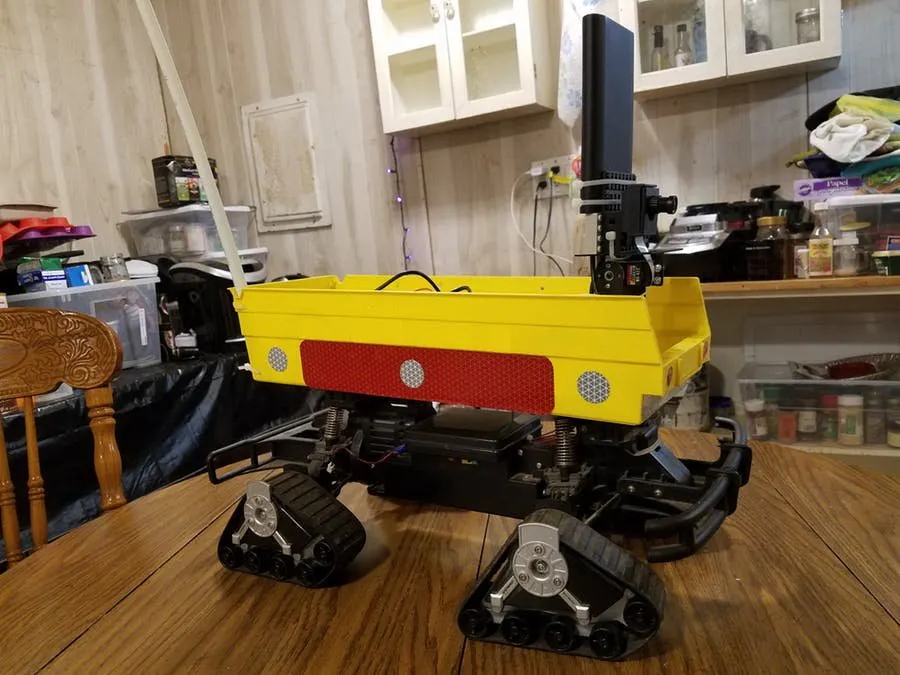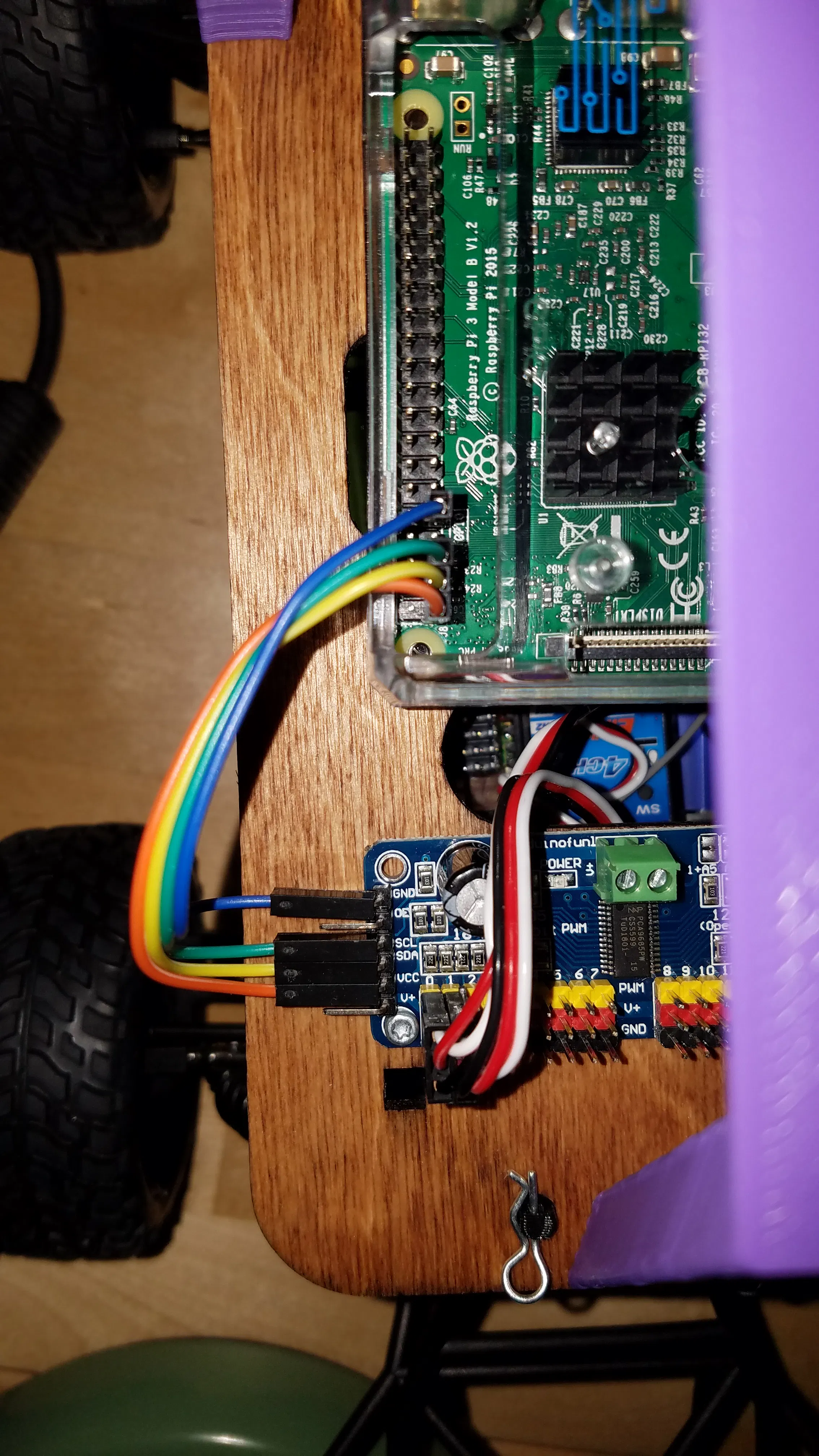Created June 24, 2018 © GPL3+

# Robotic Assistant with Search and Rescue Capability

This design will perform the functions of several types of service dog. AI and sensors will provide emergency search and rescue.skills.## Things used in this project

### Hardware componentsRaspberry Pi 3 Model B
×1
 Exceed RC car
×1
 servo PWM driver board
×1

## Schematics

### RPi DonkeyCar connections

How to connect the Rpi to the PWM servo controller board## Code

### mmult.cpp with logistic function added Test1

C/C++
replaces mmult.cpp in Xilinx matrix multiply example project
```#include <stdio.h>
#include <stdlib.h>

#include "mmult.h"
// HLS Math Functions
//#include "hls_math.h"// HLS Math Functions
#include <math.h>
/**
*
* Design principles to achieve II = 1
* 1. Stream data into local RAM for inputs (multiple access required)
* 2. Partition local RAMs into N/2 sub-arrays for fully parallel access (dual-port read)
* 3. Pipeline the dot-product loop, to fully unroll it
* 4. Separate multiply-accumulate in inner loop to force two FP operators
*
*/
void mmult_accel(float A[N*N], float B[N*N], float C[N*N])
{
float _A[N][N], _B[N][N];
#pragma HLS array_partition variable=_A block factor=8 dim=2
#pragma HLS array_partition variable=_B block factor=8 dim=1

for(int i=0; i<N; i++) {
for(int j=0; j<N; j++) {
#pragma HLS PIPELINE
_A[i][j] = A[i * N + j];
_B[i][j] = B[i * N + j];
}
}

for (int i = 0; i < N; i++) {
for (int j = 0; j < N; j++) {
#pragma HLS PIPELINE
float result = 0;
for (int k = 0; k < N; k++) {
float term = _A[i][k] * _B[k][j];
result += term;
}
C[i * N + j] = 1.0/(1.0 + /*hls::*/expf(-result));
}
}
}
```

### main.cpp for logistic function Test 1

C/C++
replaces main.cpp in the Xiliinx matrix multiply example project. This adds the logistic function where it would be in neural network calculations
```#include <iostream>
#include <stdlib.h>
#include <stdint.h>

#include "sds_lib.h"
#include "mmult.h"

// Non-HLS Math for benchmark purposes (non-accelerated versions of functions)
#include <math.h>

// Fixed point support experiments soon
//#include <ap_fixed.h>

#define NUM_TESTS 1024

class perf_counter
{
public:
uint64_t tot, cnt, calls;
perf_counter() : tot(0), cnt(0), calls(0) {};
inline void reset() { tot = cnt = calls = 0; }
inline void start() { cnt = sds_clock_counter(); calls++; };
inline void stop() { tot += (sds_clock_counter() - cnt); };
inline uint64_t avg_cpu_cycles() { return (tot / calls); };
};

static void init_arrays(float *A,  float *B, float *C_sw, float *C)
{
for (int i = 0; i < N; i++) {
for (int j = 0; j < N; j++) {
A[i * N + j] = 1+i*N+j;
B[i * N + j] = rand() % (N * N);
C_sw[i * N + j] = 0.0;
C[i * N + j] = 0.0;
}
}
}

void mmult_golden(float *A,  float *B, float *C)
{
for (int row = 0; row < N; row++) {
for (int col = 0; col < N; col++) {
float result = 0.0;
for (int k = 0; k < N; k++) {
result += A[row*N+k] * B[k*N+col];
}
C[row*N+col] = 1.0/(1.0 + expf(-result)); // non-accelerated logistic function
}
}
}

static int result_check(float *C, float *C_sw)
{
for (int i = 0; i < N * N; i++) {
if (C_sw[i] != C[i]) {
std::cout << "Mismatch: data index=" << i << "d=" << C_sw[i]
<< ", dout=" << C[i] << std::endl;
return 1;
}
}
return 0;
}

int mmult_test(float *A,  float *B, float *C_sw, float *C)
{
std::cout << "Testing " << NUM_TESTS << " iterations of " << N << "x" << N
<< " floating point mmult..." << std::endl;

perf_counter hw_ctr, sw_ctr;

for (int i = 0; i < NUM_TESTS; i++)
{
init_arrays(A, B, C_sw, C);

sw_ctr.start();
mmult_golden(A, B, C_sw);
sw_ctr.stop();

hw_ctr.start();
mmult_accel(A, B, C);
hw_ctr.stop();

if (result_check(C, C_sw))
return 1;
}
uint64_t sw_cycles = sw_ctr.avg_cpu_cycles();
uint64_t hw_cycles = hw_ctr.avg_cpu_cycles();
double speedup = (double) sw_cycles / (double) hw_cycles;

std::cout << "Average number of CPU cycles running mmult in software: "
<< sw_cycles << std::endl;
std::cout << "Average number of CPU cycles running mmult in hardware: "
<< hw_cycles << std::endl;
std::cout << "Speed up: " << speedup << std::endl;

return 0;
}

/**
* Design principles to achieve performance
*
* 1. sds_alloc to guarantee physically contiguous buffer allocation
*    that enables the most efficient DMA configuration (axidma_simple)
*/
int main(int argc, char* argv[]){
int test_passed = 0;
float *A, *B, *C_sw, *C;

A = (float *)sds_alloc(N * N * sizeof(float));
B = (float *)sds_alloc(N * N * sizeof(float));
C = (float *)sds_alloc(N * N * sizeof(float));
C_sw = (float *)sds_alloc(N * N * sizeof(float));

if (!A || !B || !C || !C_sw) {
if (A) sds_free(A);
if (B) sds_free(B);
if (C) sds_free(C);
if (C_sw) sds_free(C_sw);
return 2;
}

test_passed = mmult_test(A, B, C_sw, C);

std::cout << "TEST " << (test_passed ? "FAILED" : "PASSED") << std::endl;

sds_free(A);
sds_free(B);
sds_free(C);
sds_free(C_sw);

return (test_passed ? -1 : 0);
}
```

## Credits

### Tom Minnich

17 projects • 69 followers
Embedded software guy for a long time